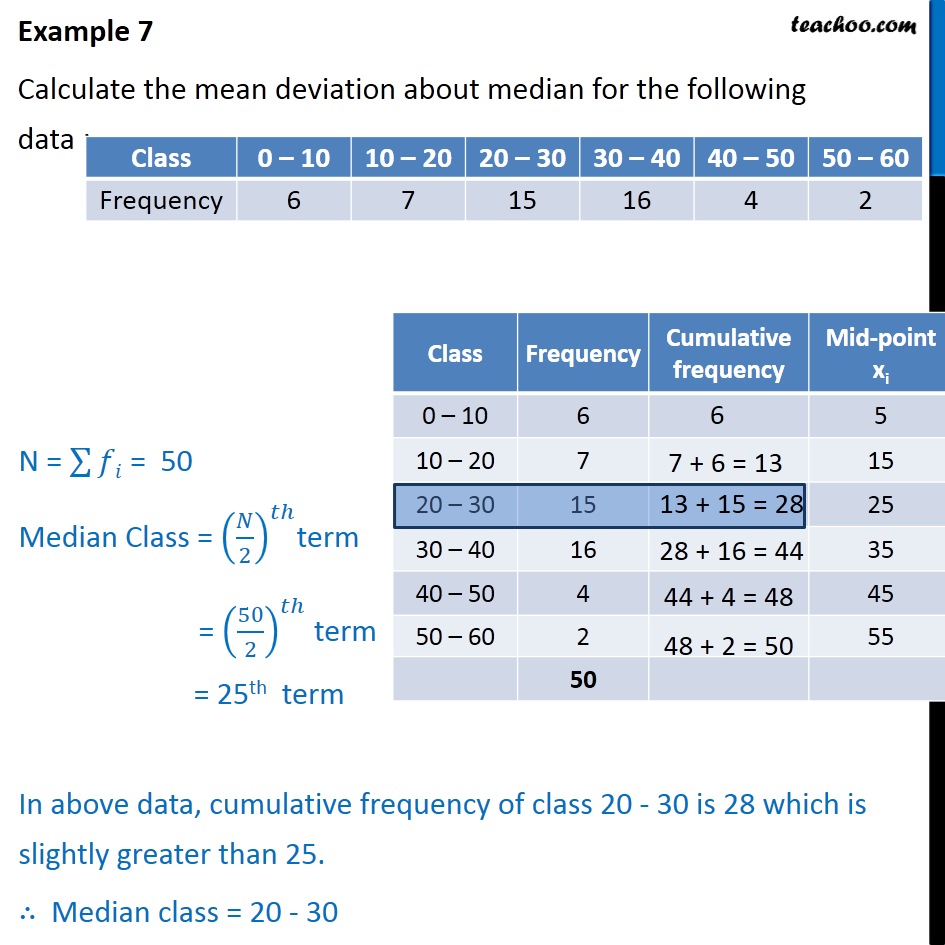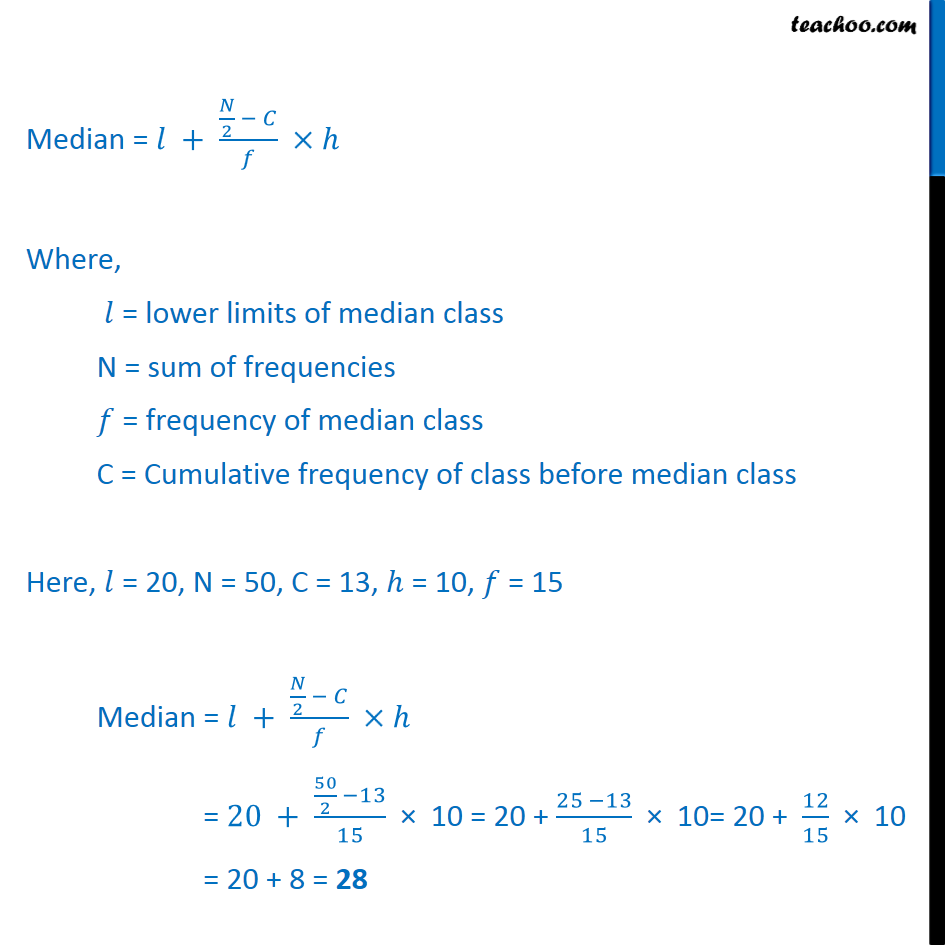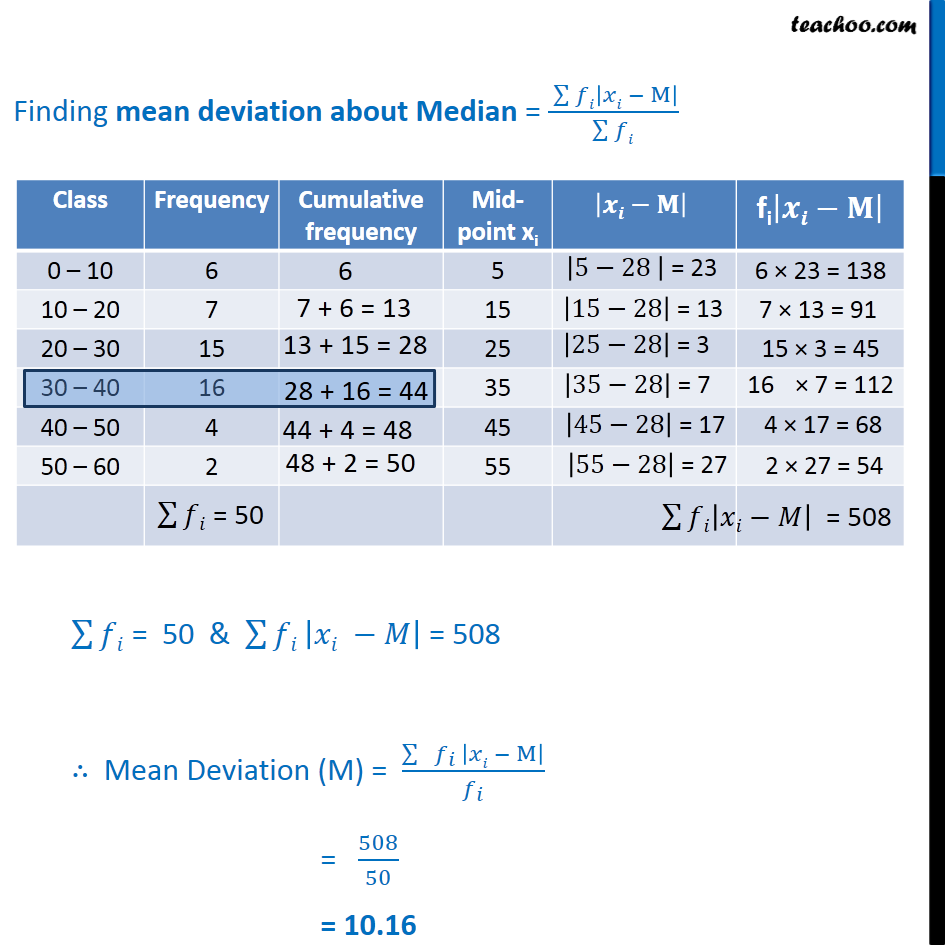Examples

Chapter 13 Class 11 Statistics
Serial order wiseLearn in your speed, with individual attention - Teachoo Maths 1-on-1 Class

### Transcript

Example 7 Calculate the mean deviation about median for the following data : Median = 𝑙 + 𝑁﷮2﷯ − 𝐶﷮𝑓﷯ ×ℎ Where, 𝑙 = lower limits of median class N = sum of frequencies 𝑓 = frequency of median class C = Cumulative frequency of class before median class Here, 𝑙 = 20, N = 50, C = 13, ℎ = 10, 𝑓 = 15 Median = 𝑙 + 𝑁﷮2﷯ − 𝐶﷮𝑓﷯ ×ℎ = 20 + 50﷮2﷯ −13﷮15﷯ × 10 = 20 + 25 −13﷮15﷯ × 10= 20 + 12﷮15﷯ × 10 = 20 + 8 = 28 Finding mean deviation about Median = ﷮﷮𝑓𝑖 𝑥𝑖 − M﷯﷯﷮ ﷮﷮𝑓𝑖﷯﷯